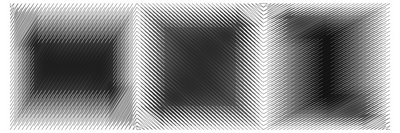Three Faces of PiFractals aren’t just shapes; fractals can be numbers as well. A fractal sequence is one that, in some sense, contains infinite copies of itself. One example of a fractal sequence is the signature of an irrational number (a number that can’t be expressed as a fraction, like π or the square root of 2). To create a fractal signature sequence for a number x, create values of y, where y = i + jx and i and j are both integers. Create y values for i and j from 1 to infinity and order them from smallest to largest. If x is irrational, then every y value will be different and the sequence of the i’s and the sequence of the j’s are both fractal sequences.

Infinity is really big, so instead, let i and j both vary from 0 to 32. Then, draw a line from (0, 0) to (32, 32), going through each point in order of the y values. Different irrational numbers will create different graphs. Even though each graph is one continuous line, the angles of the zigs and zags change, giving the appearance of rectangular blocks of different shades of gray.

The above image is composed of three separate graphs, aligned so that the entire image is one continuous line. The graphs represent three different irrational numbers which are based on π: π/2 (~ 1.5708), the natural logarithm of π (~1.145), and the square root of π (~1.772).